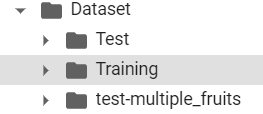# ImageDataBunch.from_folder() error

I am trying to use the Fruits-360 Dataset from Kaggle. It looks like following:Training folder has various sub folders of different fruits. Same goes for test folder.
So what I want to do is use the data_block API. So I tried doing following things:

Edit: Path is defined like this:

``````base_dir = root_dir + '/Colab Notebooks/Dataset'
path = Path(base_dir)
``````

1.` data = ImageDataBunch.from_folder(path=path, train=‘Training’, valid=‘Test’, size=image_size, bs=batch_size, num_workers=0)

``````But it is giving me following error:
``````

`IndexError: index 0 is out of bounds for axis 0 with size 0`

I tried the solutions suggested in the similar question. Like following:
data = (ImageList.from_folder(path)
.split_by_random_pct()
.label_from_folder()
.transform(tfms, size=image_size)
.databunch()
)
But it still doesnt work?

Also, I want to split the training folder itself into train and validation sets. But it is still giving the same error.

What is it that I am doing wrong? Thank You.

Can you help please ? @TomB @cwerner @sgugger